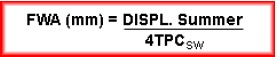# What is Fresh Water Allowance (FWA) and its formula?

Fresh Water Allowance (FWA) is the number of millimetres by which the mean draught changes when a ship passes from salt water to fresh water, or vice-versa, when the ship is loaded to the Summer displacement.

The FWA is found by the formula: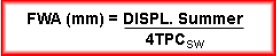TPCSW is the salt-water TPC value for the summer load draught.

Derivation of FWA:

Displacement of a ship may be calculated by = L x B x d x Cb x ρ

Consider the ship shown loaded to the Summer draught floating in:

(i) salt water;

(ii) fresh water.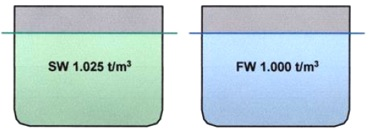The displacements will be different but the volumes of displacement will be the same. If a ship is floating at a draught in FW, to find the equivalent SW displacement for the same draught:

DISPLSW = DISPLFW x 1.025

Therefore, for the ship shown:

DISPLFW  x 1.025 = DISPLFW + (WPA x FWA x 1.000 t/m3)

Where, FWA is expressed in meters.

DISPLFW x 1.025 = DISPLFW + (WPA x FWA)

1.025 DISPLFW = DISPLFW + (WPA x FWA)

1.025 DISPLFW – DISPLFW = (WPA x FWA)

0.025 DISPLFW = (WPA x FWA) (I)

&nbsp

;

Consider now the formula for TPC, where the TPCSW is the weight required to sink the ship whilst at the summer displacement by 1 cm:

TPCSW = WPA x 1.025/100

Rearranging this gives:

WPA = TPCSW/100 x 100        (ii)

Where,  the WPA is that for the Summer load draught waterline.

Substituting equation (ii) into equation (i):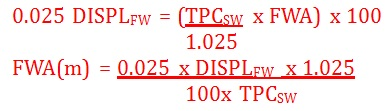To express FWA in mm then: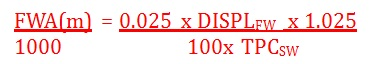Rearranging gives: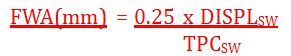Therefore: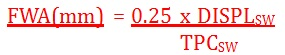[contentcards url=”https://5gplans.co.in/2021/05/28/bharti-airtel-hyperfast-5g-plans-and-launch-date/”]

Thus: